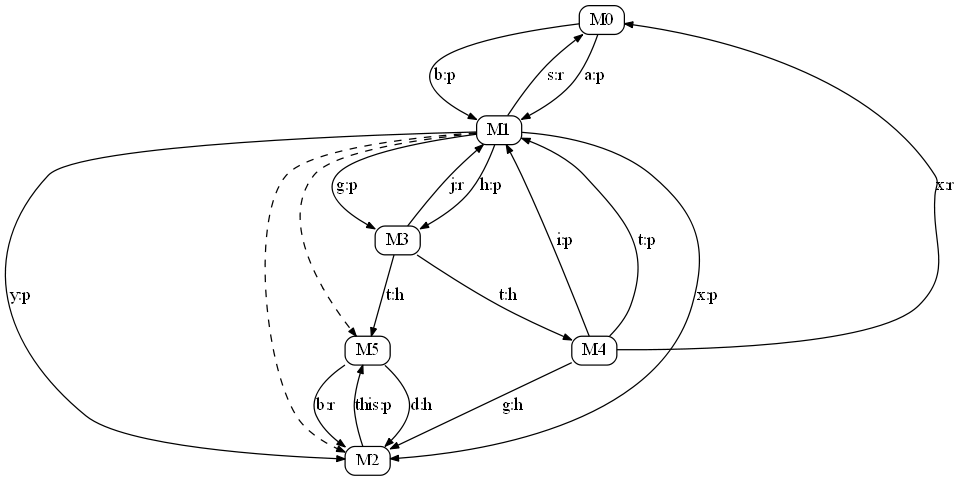# Illustration of the MDG

The Method-Dependence Graph (MDG) models all data and control dependencies but directly at method level. We illustrate this lightweight dependence model on this page with an example Java program and its MDG to be used by impact analysis techniques and other client analyses.

## Example Program

```
/* example code for MDG */
public class A {
static int g; public int d;
String M1(int f, int z) {
int x = f + z, y = 2, h=21;
if (x > y) {
M2(x,y);
}
int r = new B().M3(h,g);
String s = "M3 retval: " + r;
return s;
}

void M2(int m, int n) {
int t = m - d;
if (t > 0) {
n = g/t;
}
boolean b = C.M5(this);
//System.out.println(b);
}
}

/* example code for MDG */
public class B {
static short t;

int M3(int a, int b) {
int j = 0;
t = -250;

if ( a < b ) {
j = b - a;
}
return j;
}

static double M4() {
int x = A.g, i = 5;

try {
A.g = x/(i+t);
new A().M1(i, t);
}
catch(Exception e) {	}

return x;
}
}

/* example code for MDG --- this is the entry class */
public class C {
}

public static boolean M5(A q) {
long y = q.d;
boolean b = B.t > y;
q.d = 2;
return b;
}

public static void main(String[] args) {
int a = 0, b = -3;
String s = new A().M1(a,b);
double d = B.M4();
String u = s + d;
System.out.println(u);
}
}

```

## the MDG of the Example Program

Data-dependence edges are labeled with the associated variables and the edge types separated with a colon. The data-dependence edge types are:

• p -- parameter edge
• r -- return edge
• h -- heap edge

Control-dependence edges are not labeled. Yet, line styles are used in this illustration to distinguish data and control dependencies:

• solid line -- interprocedural data dependence edge
• dashed line -- interprocedural control dependence edgeNote:
This diagram is a conceptual illustration only;
The real MDG that out approach built and used for impact analysis is based on the Soot Jimple IR (an immediate representation of Java code transformed from the Java byte-code).

Generated on 4 Apr 2015 by1.6.1